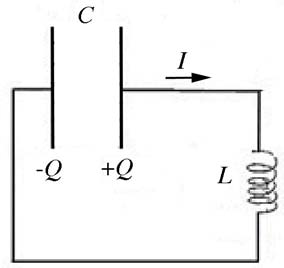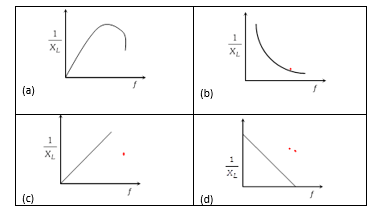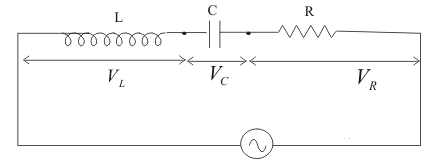# Alternating Current Important Questions

Question 1
Why it is not possible to have electrolysis by A.C?

Question 2
What is the value of the inductive reactance XL in a DC circuit?

## Multiple Choice Questions

Question 3
Which of the these works on the principle of self-induction?
(a) Transformer
(b) Transistor
(c) choke
(d) None of these

Question 4
If the velocity of the object is zero,the acceleration would also be zero
(a) True
(b) False

Question 5
What is true of any AC circuit?
(a) None of these
(b) The power dissipation happens in resistance only in AC circuit
(c) The algebraic sum of the rms voltage across the different element of the circuit is equal to the applied rms voltage
(d) The algebraic sum of the instantaneous voltage  across the different element of the circuit  is equal to the applied instantaneous voltage

Question 6
Transformers are used
(a) To step up or step down ac voltage
(b) To convert a.c to d.c
(c) To step up d.c voltage
(d) To convert d.c to a.c

Question 7
What is true of AC GENERATOR?
(a) It works on the principle of electromagnetic induction
(b) The mechanical energy of the rotation is converted into electrical energy
(c) A pair of slip rings are used  in the AC generator
(d) All the above

Question 8
An alternating voltage V=200sin300t is applied across a series combination of R=10Ω and L=800mH.
(a) The impedence of the circuit is 240.2Ω
(b) Power factor is given by .05
(c) The peak current in the circuit is .8326 A
(d) None of these

Question 9
In an series LC circuit, the voltage across  the L and C are
(a) 180 out of phase
(b) 90 out of phase
(c) are in same phase
(d) 45 out of phase

Question 10
Let A and B are the heat production of the electric heater in AC and DC of same voltage.
(a) A=B
(b) A> B
(c) A <B
(d) none of the above

Question 11
An inductor having a reactance of 25 and a resistance R gives off heat at the rate of 10W when it carries a current of 0.50A (rms). What is the impedance of the inductor?

Question 12
At the moment depicted in the LC circuit the current is non-zero and the capacitor plates are charged (as shown in the figure below). The energy in the circuit is stored(a) only in the electric field and is decreasing.
(b) only in the electric field and is constant.
(c) only in the magnetic field and is decreasing.
(d) only in the magnetic field and is constant.
(e) in both the electric and magnetic field and is constant.
(f) in both the electric and magnetic field and is decreasing.

Question 13
When an AC voltage of 220 V is applied to the capacitor C
(a) the maximum voltage between plates is 220 V.
(b) the current is in phase with the applied voltage.
(c) the charge on the plates is in phase with the applied voltage.
(d) power delivered to the capacitor is zero.

Question 14
A resistor of 50 ohm ,an inductor of 20/π H and a capacitor of 5/π μ F are connected in series to a voltage supply of 230 V-50 Hz, The impedance of the circuit is
(a) 10 ohm.
(b) 50 ohm.
(c) 100 ohm.
(d) 1000 ohm.

Question 15
Dimension of the impedance is
(a) $[M L^2 T^{-3} A^{-2}]$
(b) $[M L^2 T^{-3} A^{-1}]$
(c) $[M L^2 T^{-2} A^{-2}]$
(d) $[M L^2 T^{-1} A^{-2}]$

Question 16
In pure inductive circuit, the curves between frequency f and reciprocal of inductive reactance $\frac {1}{X_L}$ isQuestion 17
if C and R denote Capacitance and Resistance, then dimension of CR is
(a) $[M^0 L^0 T^{-1} A^{0}]$
(b) $[M^0 L^0 T^{1} A^{0}]$
(c) $[M^0 L^0 T^{1} A^{-2}]$
(d) $[M^0 L^1 T^{1} A^{0}]$

Question 18
In a AC circuit ,the current is expressed as
$I= 200 \ sin 100 \pi t$.
In this circuit , the current rises from zero to peak value in time
(a) 1/200 sec
(b) 1/100 sec
(c) 1/300 sec
(d) 1/400 sec

Question 19 In LCR series circuit connected with an AC voltage source of frequency f, the current leads the voltage by 45°, The value of C is
(a) $\frac {1}{\pi f (2 \pi fL -R)}$
(b) $\frac {1}{2\pi f (2 \pi fL -R)}$
(c) $\frac {1}{\pi f (2 \pi fL +R)}$
(d) $\frac {1}{2\pi f (2 \pi fL +R)}$

Question 20
Quantity that remains unchanged in a transformer is
(a) Voltage
(b) Current
(c) Frequency
(d) none of these

## Text Based Questions

Question 1
Show that in the free oscillations of an LC circuit, the sum of energies stored in the capacitor and the inductor is constant in time.
Question 2
What is the principle of transformer? . Explain how laminating the core of a transformer helps to reduce eddy current losses in it. Why the primary and secondary coils of a transformer are preferably wound on the same core

Question 3
A lamp is connected in series with a capacitor. Predict your observations for dc and ac connections. What happens in each case if the capacitance of the capacitor is reduced?

Question 4
Prove that an ideal capacitor in an A.C circuit does not dissipate power?
Question 5
(i) Explain briefy with the help if labelled diagram , the basic principle of the working of an AC generator
(ii) In an A.C generator , coil of N turns and Area A is rotataed at 'v' revolutions per sec in a uniform magnetic field B. Write the expression for the EMF produced
Question 6
(i) Derive the expression for the average power consumed in a series LCR circuit connected to AC source where phase difference between voltage and current is φ
(ii) Define the quality factor in an AC circuit?
Question 7
Why is choke coil neeed in the use of fluorescent tubles with AC mains?

## Numerical Questions

Question 1
A 100 μF capacitor in series with a 40 &Omega resistance is connected to 100 V 60Hz supply
Calculate the following
a. Reactance
b. Impedance
c. Maximum Current in the circuit
d. rms voltage across the resistor and capacitor.Is the algebraic sum of these voltages more than the source voltage?. if Yes resolve the paradox

Question 2
An A.C voltage given by $V= 70 \sin 100 \pi t$ is connected across a pure resistor of 25 Ω. Find the following
a. Frequency of the source
b. rms current through the resistor

Question 3
An inductor L of inductance $X_L$ is connected in series with a bulb B and an ac source.
How would brightness of the bulb change when (i) number of turn in the inductor is reduced, (ii) an iron rod is inserted in the inductor and (iii) a capacitor of reactance $X_C = X_L$ is inserted in series in the circuit. Justify your answer in each case.

Question 4
The given circuit diagram shows a series LCR circuit connected to a variable frequency 230 V source. Here L=5.0 H, C=80 μ F, R=40 Ω(a) Determine the source frequency, which drives the circuit in resonance.
(b) Obtain the impedance of the circuit and the amplitude of current at the resonating frequency.
(c) Determine the rms potential drops across the three elements of the circuit.
(d) How do you explain the observation that the algebraic sum of the voltage of the three elements obtained in (c) is greater than the supplied voltage?

Question 5
A pure inductor of 25.0 mH is connected to a source of 220 V. Find the inductive reactance and rms current in the circuit if the frequency of the source is 50 Hz.